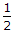# Electronics - Resistance and Power

### Exercise :: Resistance and Power - General Questions

21.

One problem with mechanically variable resistors is noticeable in audio circuits as:

 A. scratchy noise B. lack of bass response C. variable volume D. too much treble response

Explanation:

No answer description available for this question. Let us discuss.

22.

A color code of orange, orange, orange is for what ohmic value?

 A. 22 kilohms B. 3300 ohms C. 44000 ohms D. 33 kilohms

Explanation:

No answer description available for this question. Let us discuss.

23.

A conductor's cross-sectional area in circular mils forinch is:

 A. 500 cmils B. 100,000 cmils C. 1,000,000 cmils D. 500,000,000 cmils

Explanation:

No answer description available for this question. Let us discuss.

24.

If a variable resistor's resistance varies in a nonuniform manner as the shaft is moved, it is considered to be:

 A. linear B. defective C. not wirewound D. tapered

Explanation:

No answer description available for this question. Let us discuss.

25.

Power is measured in units of:

 A. joules x charge B. joules/work C. joules x voltage D. joules/time